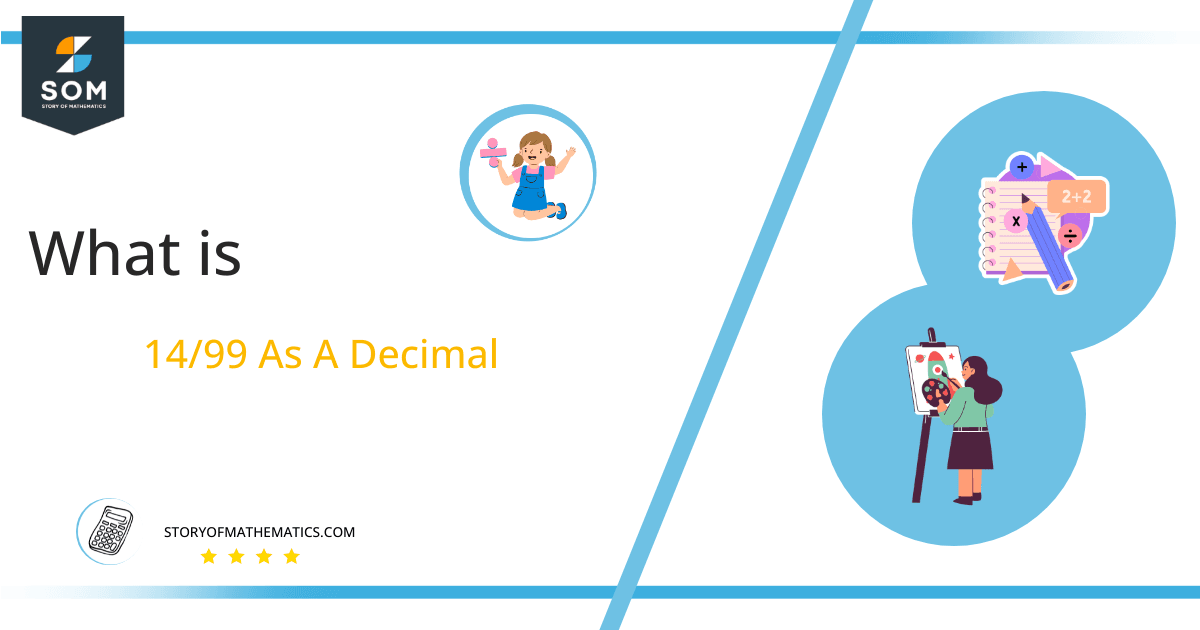# What Is 14/99 as a Decimal + Solution With Free Steps

The fraction 14/99 as a decimal is equal to 0.141.

The fraction 14/99 is a recurring decimal fraction. It can be converted into decimal representation by using the division method. In decimal notation, its digits are repeating a group of terms at regular intervals.

Here, we are more interested in the division types that result in a Decimal value, as this can be expressed as a Fraction. We see fractions as a way of showing two numbers having the operation of Division between them that result in a value that lies between two Integers.Now, we introduce the method used to solve said fraction to decimal conversion, called Long Division, which we will discuss in detail moving forward. So, let’s go through the Solution of fraction 14/99.

## Solution

First, we convert the fraction components, i.e., the numerator and the denominator, and transform them into the division constituents, i.e., the Dividend and the Divisor, respectively.

This can be done as follows:

Dividend = 14

Divisor = 99

Now, we introduce the most important quantity in our division process: the Quotient. The value represents the Solution to our division and can be expressed as having the following relationship with the Division constituents:

Quotient = Dividend $\div$ Divisor = 14 $\div$ 99

This is when we go through the Long Division solution to our problem. The following figure shows the solution for fraction 14/99.Figure 1

## 14/99 Long Division Method

We start solving a problem using the Long Division Method by first taking apart the division’s components and comparing them. As we have 14 and 99, we can see how 14 is Smaller than 19, and to solve this division, we require that 14 be Bigger than 99.

This is done by multiplying the dividend by 10 and checking whether it is bigger than the divisor or not. If so, we calculate the Multiple of the divisor closest to the dividend and subtract it from the Dividend. This produces the Remainder, which we then use as the dividend later.

Now, we begin solving for our dividend 14, which after getting multiplied by 10 becomes 140.

We take this 140 and divide it by 99; this can be done as follows:

140 $\div$ 99 $\approx$ 1

Where:

99 x 1 = 99

This will lead to the generation of a Remainder equal to 140 – 99 = 41. Now this means we have to repeat the process by Converting the 41 into 410 and solving for that:

410 $\div$ 99 $\approx$ 4

Where:

99 x 4 = 396

This, therefore, produces another Remainder which is equal to 410 – 396 = 14. Now this means we have to repeat the process by Converting the 14 into 140 and solving for that:

140 $\div$ 99 $\approx$ 1

Where:

99 x 1 = 99

Finally, we have a Quotient generated after combining the three pieces of it as 0.141, with a Remainder equal to 41.Images/mathematical drawings are created with GeoGebra.